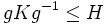# Variety-dominating subgroup

## Contents

BEWARE! This term is nonstandard and is being used locally within the wiki. [SHOW MORE]
This article defines a subgroup property: a property that can be evaluated to true/false given a group and a subgroup thereof, invariant under subgroup equivalence. View a complete list of subgroup properties[SHOW MORE]

## Definition

A subgroup$H$ of a group$G$ is termed variety-dominating if there exists a subvariety$\mathcal{V}$ of the variety of groups such that:

•$H \in \mathcal{V}$.
• If$K \le G$ is such that$K \in \mathcal{V}$, there exists$g \in G$ such that$gKg^{-1} \le H$.